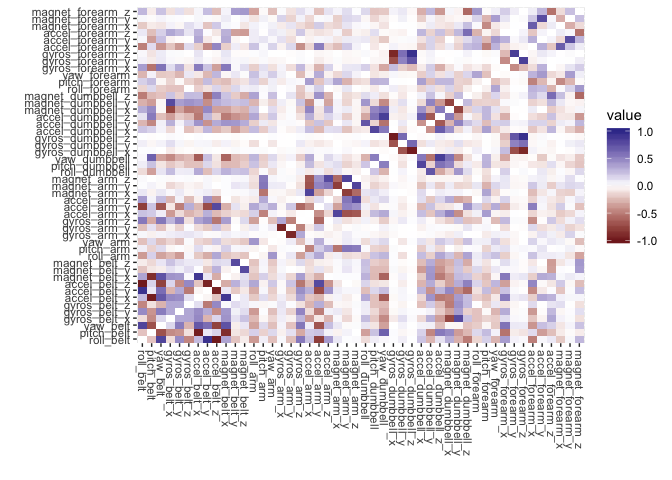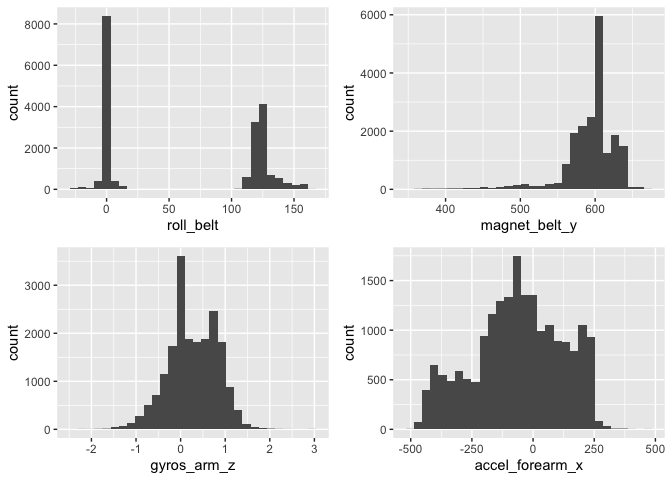## Introduction

In this investigation, I try to classify the manner in which a physical exercise was done using accelerometer, gyroscope and magnetometer data. In an experiment, under expert supervision, six participants performed the same exercise (a bicep curl) in the correct fashion and four common mistakes.

These mistakes included throwing elbows to the front, lifting the dumbbell only halfway, lowering the dumbbell only halfway, and throwing the hips to the front. Data was collected from three instruments on the participant’s belt, forearm, arm, and dumbbell.

Being able to classify the manner in which an exercise was completed, rather than just the quantity of exercises finished, would have a number of important applications related to exercise safety and performance.

The given task is really focused only on prediction, and so the tradeoffs we associate with achieving greater accuracy (reduced scalability, interpretability, speed or simplicity) can be ignored in favor of better predictions.

In this cases, making any particular kind of misclassification error is no more damaging than another so I won’t need to make a certain kind of mistake more costlier than others. That is, we can treat false negatives and false positives equally. Overall accuracy, rather than the sensitivity or specificity, will be the guiding metric.

## Data Preparation

``````# load libraries
library(caret)
library(plyr)
library(dplyr)
library(reshape2)
library(ggplot2)
library(gridExtra)
library(class)
library(gmodels)
library(naivebayes)
library(C50)
library(rpart)
library(randomForest)``````

First, I need to read in the data. Note that I’ve called the provided testing set the validation set, as I’ll have data sampled from the training set that I’ll refer to as the testing data.

``````# download and read in training if not present
if (!file.exists("training.csv")) {
url <- "https://d396qusza40orc.cloudfront.net/predmachlearn/pml-training.csv"
}

if (!exists("trainingRaw")) {
}

if (!file.exists("testing.csv")) {
url <- "https://d396qusza40orc.cloudfront.net/predmachlearn/pml-testing.csv"
}

if (!exists("testing")) {
}``````

After reading in the data, I have an initial training set of 19622 rows and 160 columns, one of which is the outcome variable, `classe`, that we want to predict. The validation set is only 20 observations.

### Handle Missing Data

Missing data is a problem for many of the machine learning algorithms I’d like to run to build my models, and one of the first things to notice when looking at this training set is the prevalence of missing data. Out of 19622 observations, we have only 406 complete cases.

``````# instances of missing data in each column in training set
missingCols <- cbind(colSums(is.na(trainingRaw)))

# samples of complete and missing predictors
cbind(missingCols[1:5,])``````
``````##                      [,1]
## X                       0
## user_name               0
## raw_timestamp_part_1    0
## raw_timestamp_part_2    0
## cvtd_timestamp          0``````
``cbind(missingCols[27:32,])``
``````##                       [,1]
## var_total_accel_belt 19216
## avg_roll_belt        19216
## stddev_roll_belt     19216
## var_roll_belt        19216
## avg_pitch_belt       19216
## stddev_pitch_belt    19216``````

Looking at the missing data column-wise, we can see two types of columns with regard to missing data in the training set: 93 columns fully complete and 67 columns each missing 1.921610^{4} observations.

Rather than trying a strategy like median or k-nearest neighbors imputation on the missing data, it is important to realize that these columns actually represent summary statistics for the other columns, for instance, columns beginning with `avg`, `stddev` or `var`. These summary statistics correspond to the binary `new_window` variable, and so we can be confident in removing these columns (as well as `new_window`) from consideration in training and validation sets.

• (As a side note, if we were interested in interpretability, it may be interesting to try to build a model only with the summary statistics.)

Because I have been tasked with making predictions from only the measurements, I’ll also remove the first few predictor variables including some of the time series data.

``````# find summary predictors
sum_stats <- c("max","min","amplitude","avg","stddev","kurtosis",
"skewness","total", "var")
drops <- unique(grep(paste(sum_stats, collapse = "|"),
names(trainingRaw), value = TRUE))

# find other unnecessary predictors
non_numeric_cols <- c("X", "user_name", "raw_timestamp_part_1",
"raw_timestamp_part_2","cvtd_timestamp","new_window",
"num_window")

# compete list of columns to be dropped
drops <- append(drops, non_numeric_cols)

# drop columns in training and validation sets
training <- trainingRaw[ , !(names(trainingRaw) %in% drops)]
validation <- validation[ , !(names(validation) %in% drops)]``````

### Checking Data Types

Having reduced the number of predictor variables from 159 to 159. The `str()` function shows that some predictors are stored as numeric, while others are integer. I’ll make them all numeric.

``````# convert measurements to numeric variables
training[,1:48] <- lapply(training[,1:48], as.numeric)
validation[,1:48] <- lapply(validation[,1:48], as.numeric)``````

### Check for Near Zero Variance Predictors

After removing columns with missing data, we can check for predictor variables with zero or near zero variance because these would likely be poor predictors. It returns 0 rows so we have no concerns from this perspective.

``````# calculate zero and near zero variance predictors
nearzero <- nearZeroVar(training, saveMetrics = TRUE)
nrow(nearzero[nearzero\$nzv == TRUE,])``````
``##  0``

### Check for Multicollinearity

I should also check the predictor variables for multicollinearity. I can do this with a correlation matrix.

``````# create correlation matrix
cor.matrix <- cor(training[,-49])

# set correlations of variables with themselves to 0
diag(cor.matrix) <- 0

# plot correlation matrix
ggplot(melt(cor.matrix), aes(x = Var1, y = Var2, fill = value)) +
geom_tile() +
theme(axis.text.x = element_text(angle=-90, vjust=0.5, hjust=0)) +
labs(x = "", y = "")``````I can see the most highly correlated variables in a table.

``````# find most highly correlated predictors
high_cors <- filter(arrange(melt(cor.matrix), -abs(value)), abs(value) > 0.8)
high_cors[-seq(0, nrow(high_cors), 2),]``````
``````##                Var1             Var2      value
## 1      accel_belt_z        roll_belt -0.9920085
## 3  gyros_dumbbell_z gyros_dumbbell_x -0.9789507
## 5      accel_belt_x       pitch_belt -0.9657334
## 7      accel_belt_z     accel_belt_y -0.9333854
## 9   gyros_forearm_z gyros_dumbbell_z  0.9330422
## 11     accel_belt_y        roll_belt  0.9248983
## 13      gyros_arm_y      gyros_arm_x -0.9181821
## 15  gyros_forearm_z gyros_dumbbell_x -0.9144764
## 17    magnet_belt_x     accel_belt_x  0.8920913
## 19    magnet_belt_x       pitch_belt -0.8841727
## 21 accel_dumbbell_z     yaw_dumbbell  0.8491322
## 23  gyros_forearm_z  gyros_forearm_y  0.8455626
## 25         yaw_belt        roll_belt  0.8152297
## 27     magnet_arm_z     magnet_arm_y  0.8144455
## 29     magnet_arm_x      accel_arm_x  0.8142732
## 31 accel_dumbbell_x   pitch_dumbbell  0.8082885``````

Some variables do appear to be highly correlated. We have 16 pairs of variables with a correlation above 0.8.

Because my goal is only prediction, as opposed to interpretation, there is not too much to gain by trying to remove further variables from our model. Without a better understanding of the underlying physics, the reasons for these correlations are not immediately apparent.

### Predictor Summary

To summarize our 48 predictors, we have three instruments (accelerometer, gyroscope and magnetometer) taking measurements in four places (belt, arm, forearm, and dumbbell) plus the roll, pitch and yaw measurements for each.

``````# predictor variables
names(training[,1:48])``````
``````##   "roll_belt"         "pitch_belt"        "yaw_belt"
##   "gyros_belt_x"      "gyros_belt_y"      "gyros_belt_z"
##   "accel_belt_x"      "accel_belt_y"      "accel_belt_z"
##  "magnet_belt_x"     "magnet_belt_y"     "magnet_belt_z"
##  "roll_arm"          "pitch_arm"         "yaw_arm"
##  "gyros_arm_x"       "gyros_arm_y"       "gyros_arm_z"
##  "accel_arm_x"       "accel_arm_y"       "accel_arm_z"
##  "magnet_arm_x"      "magnet_arm_y"      "magnet_arm_z"
##  "roll_dumbbell"     "pitch_dumbbell"    "yaw_dumbbell"
##  "gyros_dumbbell_x"  "gyros_dumbbell_y"  "gyros_dumbbell_z"
##  "accel_dumbbell_x"  "accel_dumbbell_y"  "accel_dumbbell_z"
##  "magnet_dumbbell_x" "magnet_dumbbell_y" "magnet_dumbbell_z"
##  "roll_forearm"      "pitch_forearm"     "yaw_forearm"
##  "gyros_forearm_x"   "gyros_forearm_y"   "gyros_forearm_z"
##  "accel_forearm_x"   "accel_forearm_y"   "accel_forearm_z"
##  "magnet_forearm_x"  "magnet_forearm_y"  "magnet_forearm_z"``````

I won’t plot all of them here, but there is a wide range of distributions. Few if any could be considered approximately normal.

``````# a few distributions of predictor variables
p1 <- ggplot(training, aes(x = roll_belt)) + geom_histogram()
p2 <- ggplot(training, aes(x = magnet_belt_y)) + geom_histogram()
p3 <- ggplot(training, aes(x = gyros_arm_z)) + geom_histogram()
p4 <- ggplot(training, aes(x = accel_forearm_x)) + geom_histogram()
grid.arrange(p1, p2, p3, p4, ncol = 2)``````Lastly, I want to check for unbalanced outcomes in the data. Having vastly more than one type of class than another will impact how we interpret the accuracy of our results. While class A is more common than the others, it does not appear different enough to affect our models in a dataset of this size.

``````# distribution of classe outcome
round(prop.table(table(training\$classe)),4)``````
``````##
##      A      B      C      D      E
## 0.2844 0.1935 0.1744 0.1639 0.1838``````

It is a bit difficult to visualize all of the data. We could make a series of scatterplot matrices like the one below, but it’s not unreasonable to expect non-linear models to perform better in this task.

``````# scatterplot matrix for first 8 predictors
featurePlot(x = training[,1:8], y = training\$classe, plot = "pairs")``````